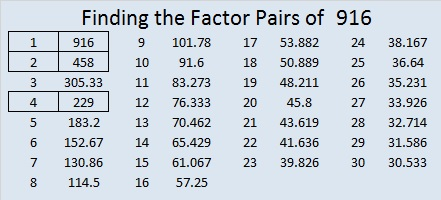# 916 Witch’s Hat

Today’s puzzle looks a little like a witch’s hat. Solving it could be bewitching. It contains a lot of perfect squares, and it’s only a level 2. Give it a try! Just make sure you don’t write a number greater than ten in the top row or the first column. Use all the numbers from one to ten in both places. There is only one solution.Print the puzzles or type the solution on this excel file: 10-factors-914-922

916 looks the same upside-down as it does right-side up, so it is a Strobogrammatic number.It looks like someone put a spell on that number!

Not only that, in BASE 26, our 916 looks like 196. There is a whole lot of magic going on here!

30² + 4² = 916, so 916 is the hypotenuse of a Pythagorean triple:
240-884-916, which can be calculated from 2(30)(4), 30² – 4², 30² + 4².

• 916 is a composite number.
• Prime factorization: 916 = 2 × 2 × 229, which can be written 916 = 2² × 229
• The exponents in the prime factorization are 2 and 1. Adding one to each and multiplying we get (2 + 1)(1 + 1) = 3 × 2  = 6. Therefore 916 has exactly 6 factors.
• Factors of 916: 1, 2, 4, 229, 458, 916
• Factor pairs: 916 = 1 × 916, 2 × 458, or 4 × 229
• Taking the factor pair with the largest square number factor, we get √916 = (√4)(√229) = 2√229 ≈ 30.2654919